##### Pre-Calculus: 1001 Practice Problems For Dummies (+ Free Online Practice)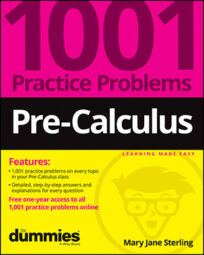Trigonometric functions are special in several ways. The first characteristic that separates them from all the other types of functions is that input values are always angle measures. You input an angle measure, and the output is some real number.

The angle measures can be in degrees or radians — a degree being one-360th of a slice of a circle, and a radian being about one-sixth of a circle. Each type has its place and use in the study of trigonometry.

Another special feature of trig functions is their periodicity; the function values repeat over and over and over, infinitely. This predictability works well with many types of physical phenomena, so trigonometric functions serve as models for many naturally occurring observations.

You’ll work with trigonometric functions and their properties in the following ways:

• Defining the basic trig functions using the sides of a right triangle

• Expanding the input values of trig functions by using the unit circle

• Exploring the right triangle and trig functions to solve practical problems

• Working with special right triangles and their unique ratios

• Changing angle measures from degrees to radians and vice versa

• Determining arc length of pieces of circles

• Using inverse trig functions to solve for angle measures

• Solving equations involving trig functions

Don’t let common mistakes trip you up; keep in mind that when working with trigonometric functions, some challenges will include the following:

• Setting up ratios for the basic trig functions correctly

• Recognizing the corresponding sides of right triangles when doing applications

• Remembering the counterclockwise rotation in the standard position of angles

• Measuring from the terminal side to the x-axis when determining reference angles

• Keeping the trig functions and their inverses straight from the functions and their reciprocals

## Practice problems

1. Use the triangle to find the trig ratio.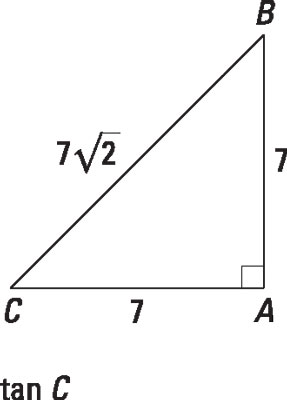Credit: Illustration by Thomson Digital

Using the right-triangle definition for the tangent of an angle, determine the measure of the side opposite the angle in question and divide that measure by the length of the side adjacent to the angle (not the hypotenuse):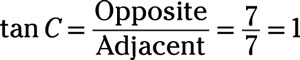Jase is in a hot air balloon that is 600 feet above the ground, where he can see his brother Willie. The angle of depression from Jase’s line of sight to Willie is 25 degrees. How far is Willie from the point on the ground directly below the hot air balloon?

The diagram shows the situation. The angle of depression is outside the triangle. However, the angle of depression is defined as the angle between a horizontal line and the observer’s line of sight. The horizontal line is parallel to the ground, so the angle of depression is equal to the angle formed by the line of sight and the ground.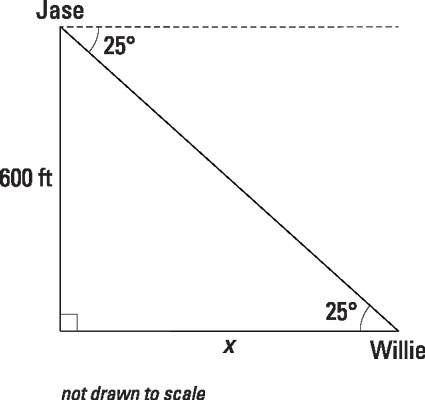Credit: Illustration by Thomson Digital

The side you’re looking for is adjacent to the given angle and the given side is opposite the angle, so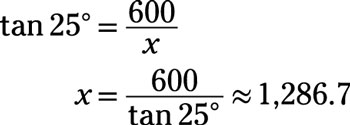Willie is about 1,286.7 feet from the point on the ground directly below the hot air balloon.